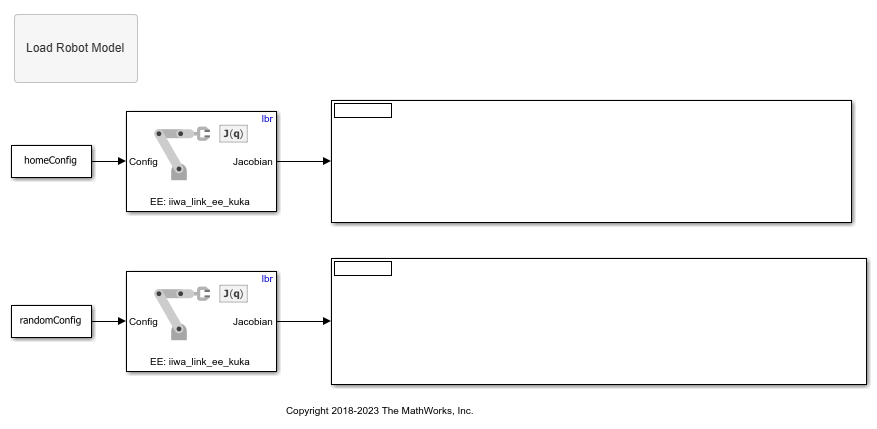# Compute Geometric Jacobian for Manipulators in Simulink

This example shows how to calculate the geometric Jacobian for a robot manipulator by using a `rigidBodyTree` model. The Jacobian maps the joint-space velocity to the end-effector velocity relative to the base coordinate frame. In this example, you define a robot model and robot configurations in MATLAB® and pass them to Simulink® to be used with the manipulator algorithm blocks.

Load a `rigidBodyTree` object that models a KUKA LBR iiwa 7 robot from the robot library. Use the `homeConfiguration` function to get the home configuration or home joint positions of the robot. Use the `randomConfiguration` function to generate a random configuration within the specified joint limits.

```lbr = loadrobot("kukaIiwa7", DataFormat="column"); homeConfig = homeConfiguration(lbr); randomConfig = randomConfiguration(lbr); ```

Open the model. If necessary, use the Load Robot Model callback button to reload the robot model and the configuration vectors. The `'tool0'` body is selected as the end-effector in both blocks.

```open_system("get_jacobian_example.slx") ```Run the model to display the Jacobian for each configuration.